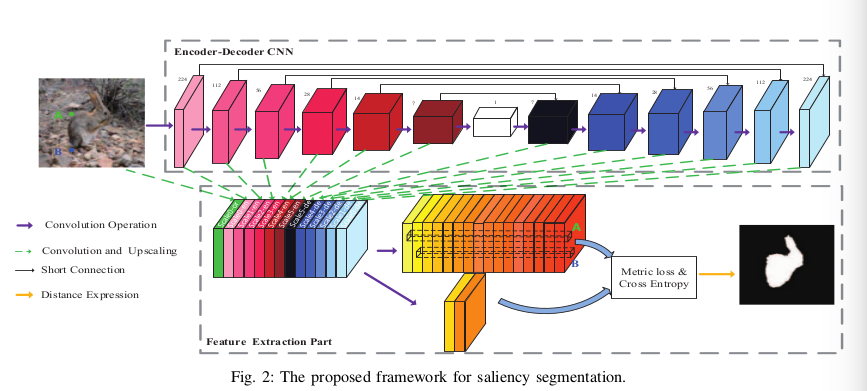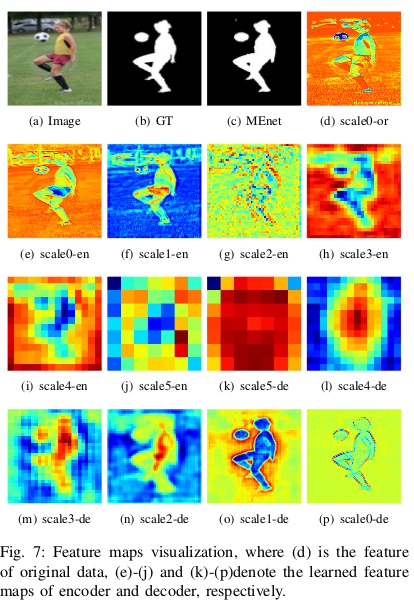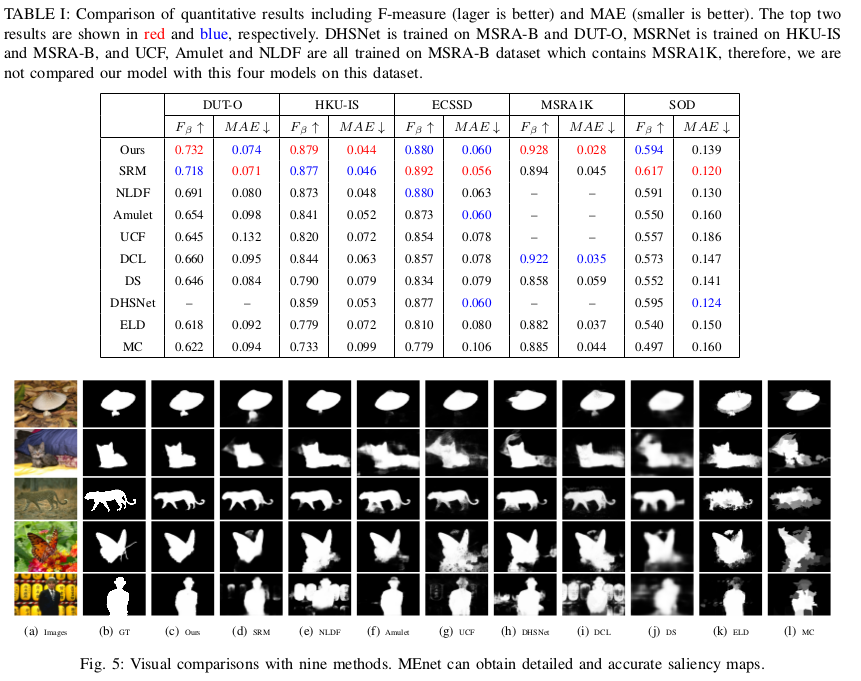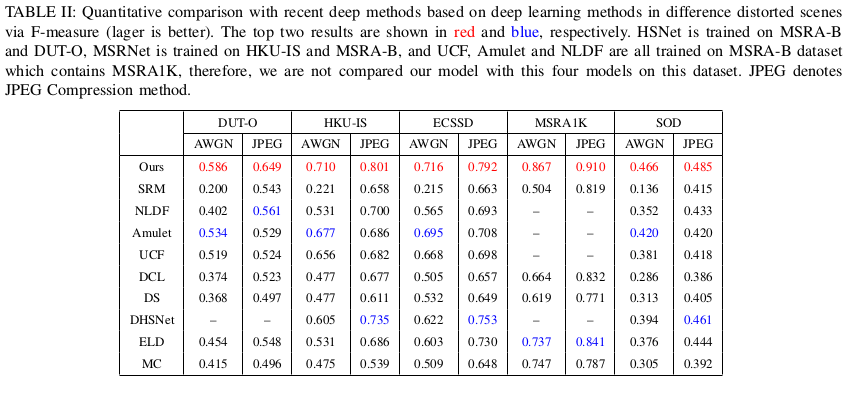This paper proposes a new architecture, a construction with a new topological metric space, with the implicit metric being determined by the deep network. Like that, they grouping all the pixels within the observed image semantically within this latent space into two regions: a salient region and a non-salient region. With this method, all feature extractions are carried out at the pixel level, which makes the output boundaries of salient object fine-grained.## New Loss

That article introduces a novel metric loss function (ML) which is based on metric learning and cross entropy.

$$L_{MEnet}(f,l|\theta)=L^{*}_{ML}(f|\theta_{2})+\lambda L_{CE}(l|\theta_{1})$$ where $$\theta = \theta_{1}\cup\theta_{2}$$, $$\lambda$$ is set to 1, $$\theta_{1}$$ is the set of learnable parameters of network to influence l and $$\theta_{2}$$ is the set of learnable parameters of network to influence f. ML is a loss function seeking to find an encoder-decoder network that enlarges the distance between any pair of feature vectors from different regions and reduces the distance from the same homogenous region by themselves.

## Experiments

Their model cost 0.086s to generate each saliency map with GPU (Titan X Pascal). The DHSNet, NLDF, Amulet, SRM, UCF and etc. need pre-trained model, and conditional random field (CRF) method is used as post-processing in DCL. Therefore, MEnet is trained from scratch.## The proposed model is robust to distorted images

To show the robustness of MEnet in the distorted setting, we work with public datasets corrupted by Additive White Gaussian Noise (AWGN) and jpeg compression (with random strengths)